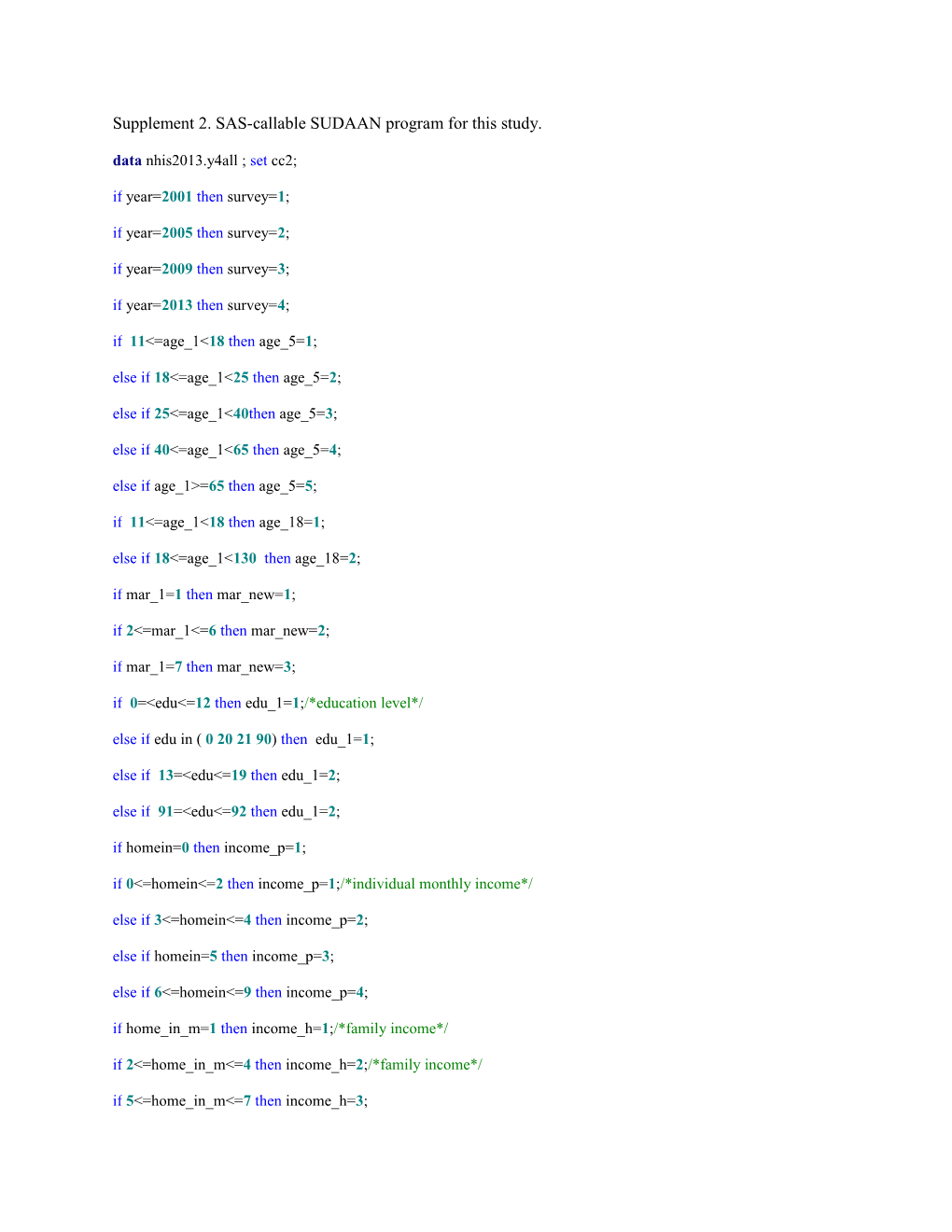# Supplement 2. SAS-Callable SUDAAN Program for This StudySupplement 2. SAS-callable SUDAAN program for this study.

data nhis2013.y4all ; set cc2;

if year=2001then survey=1;

if year=2005then survey=2;

if year=2009then survey=3;

if year=2013then survey=4;

if 11<=age_1<18then age_5=1;

elseif18<=age_1<25then age_5=2;

elseif25<=age_1<40then age_5=3;

elseif40<=age_1<65then age_5=4;

elseif age_1>=65then age_5=5;

if 11<=age_1<18then age_18=1;

elseif18<=age_1<130 then age_18=2;

if mar_1=1then mar_new=1;

if2<=mar_1<=6then mar_new=2;

if mar_1=7then mar_new=3;

if 0=<edu<=12then edu_1=1;/*education level*/

elseif edu in ( 0202190) then edu_1=1;

elseif 13=<edu<=19then edu_1=2;

elseif 91=<edu<=92then edu_1=2;

if homein=0then income_p=1;

if0<=homein<=2then income_p=1;/*individual monthly income*/

elseif3<=homein<=4then income_p=2;

elseif homein=5then income_p=3;

elseif6<=homein<=9then income_p=4;

if home_in_m=1then income_h=1;/*family income*/

if2<=home_in_m<=4then income_h=2;/*family income*/

if5<=home_in_m<=7then income_h=3;

/*define smoking status, 1:none; 2: smoker; 3:quit 2*/;

if smok in ( 012 ) then smoker=1;elseif smok in (3) and smok_now in (12) then smoker=2;

elseif smok in (3) and smok_now in (3) then smoker=3;

/* define secondhand smoking 1:none; 2: yes*/;

if year=2005 and T_smok=1then home=1;

if year=2005 and T_smok=1then oth_home=1;

if year=2005 and T_smok=1then school=1;

if year=2005 and T_smok=1then workplace=1;

if year=2005 and T_smok=1then rest=1;

if year=2005 and T_smok=1then pubic=1;

if year in (20092013) and T_smok=1then home=1 ;

if year in (20092013) and T_smok=1then oth_home=1 ;

if year in (20092013) and T_smok=1then school=1 ;

if year in (20092013) and T_smok=1then workplace=1 ;

if year in (20092013) and T_smok=1then pubic_in=1;

if year in (20092013) and T_smok=1then pubic_out=1;

if home=2 or oth_home=2 or school=2 or workplace=2then t_4_smok=2;

if home in (1) and oth_home in (1) and school in (1) and workplace in (1) then t_4_smok=1;

if year=2005 and rest in (1) and pubic in (1) then t_public_smok=1;

if year in (20092013) and pubic_in in (1) and pubic_out in (1) then t_public_smok=1;

if rest =2then t_public_smok=2;

if pubic=2then t_public_smok=2;

if pubic_in=2then t_public_smok=2;

if pubic_out=2then t_public_smok=2;

if year=2005 and t_public_smok in (12 ) then t_SHS=t_public_smok;

if year in (20092013) and pubic_in in (12) then t_SHS=pubic_in;

if smoker in (13) then smoker_now=1;/*define smoking status, 1=no*/

if smoker=2then smoker_now=2;/*define smoking status, 2=yes*/

if smoker in (2) then smoker_quit=1;/*define quit, 1=no*/

if smoker=3then smoker_quit=2;/*define quit, 2=yes*/

if smoker=1then smoker_once=1;/*define ever-smoker, 1=never*/

if smoker in (23 ) then smoker_once=2;/*define ever-smoker, 2=ever*/

if sex=3then sex=.;

if0<=smok_no<=60then smok_no_1=smok_no;

if60<smok_no<=500then smok_no_1=60;

if smoker=2 and 0<=smok_no_1<=5then smok_no_level=1;

elseif smoker=2 and 5<smok_no_1<=10then smok_no_level=2;

elseif smoker=2 and 10<smok_no_1<=20then smok_no_level=3;

elseif smoker=2 and 20<smok_no_1<=80then smok_no_level=4;

if now_UB<0then now_ub=.;

if now_ub=1then city1=1;

elseif2<=now_ub<=3then city1=2;

elseif4<=now_ub<=7then city1=3;

now_cc1=now_cc*1;

if1=<now_cc1<=7 then nowcc=now_cc1;

if now_cc1=21then nowcc=8;

if23=<now_cc1<=37 then nowcc=now_cc1-14;

if smoker in ( 12 ) then smoker_q_time=smoker;

elseif smoker in (3) and smok_qy>=1 then smoker_q_time=4;

elseif smoker in (3) and smok_qtry=2 then smoker_q_time=4;

elseif smoker in (3) then smoker_q_time=3;

/*define quit time, 1: < 1 year, 2:1 year +*/;

if smoker_q_time in ( 23 ) then smoker_q_year=1;if smoker_q_time in ( 4 ) then smoker_q_year=2;

if acl_last in (2345) then acl_y=2;

if acl_last in (01) then acl_y=acl_last;

if acl_1 in (2345) then acl_m=2;

if acl_1 in (01) then acl_m=acl_1;

if acl_1 in (2345) then acl_y=2;

if acl_1 in (01) then acl_y=1;

run;

'demographic characteristics'

Sudaan:

data kk;set nhis2013.y4all;

if age_5>1;

age_4new=age_5-1;

if1<=survey<=4;

if istrata>0;

if psu_id>0;

if sex>0;

run;

procsortdata=kk;by survey icounty istrata psu_id ; run;

proccrosstab data=kk filetype=sas design=wor;

nest survey icounty istrata psu_id;

totcnt _zero_ _zero_ psu _minus1_;

subgroup sex survey age_4new city1 edu_1 income_h income_p mar_new work_now smoker drink betel_y ;

level 2443 43232322;

tables sex*survey*(age_4new city1 edu_1 income_h mar_new work_now smoker drink betel_y);

weight wt_n;

test chisq ;

run;

Sudaan:'comparing categorical data in 2001、2005、2009、2013';

data kk;set nhis2013.y4all;

if age_5>1;

age_4new=age_5-1;

if istrata>0;

if survey>0;

if psu_id>0;

if sex>0;

run;

procsortdata=kk;by survey icounty istrata psu_id ; run;

proccrosstab data=kk filetype=sas design=wor;

nest survey icounty istrata psu_id;

totcnt _zero_ _zero_ psu _minus1_;

subgroup survey sex smoker_now smoker_quit age_4new city1 edu_1 income_h;

level 42224 323;

tables sex*age_4new*survey*(smoker_now smoker_quit);

weight wt_n;

test chisq ;

run;

Sudaan:'comparing secondhand smoking-2005、209 、2013 ';

data kk;set nhis2013.y4all;

if age_5>1;

age_4new=age_5-1;

if istrata>0;

if survey=2then survey1=3;

if survey=3then survey1=1;

if survey=4then survey1=2;

if psu_id>0;

if sex>0;

run;

procsortdata=kk;by survey icounty istrata psu_id ; run;

proccrosstab data=kk filetype=sas design=wor;

nest survey icounty istrata psu_id;

totcnt _zero_ _zero_ psu _minus1_;

subgroup smoker_now survey1 sex smoker_quit home oth_home school workplace t_public_smok t_smok;

level 2322 222222;

subpopn smoker_now=1;

tables sex*smoker_now*survey1*(home oth_home school workplace t_public_smok t_smok);

weight wt_n;

test chisq ;

run;

Sudaan:'Table 3. Factors associated with smoking in 2001 2005 2009 2013';

data kk;set nhis2013.y4all;

if age_5>1;

age_4new=age_5-1;

if istrata>0;

if psu_id>0;

if sex>0;

if smoker_now>0then smoker_now1=smoker_now-1;

run;

procsortdata=kk;by survey icounty istrata psu_id; run;

procrlogist data=kk design=wor;

nest survey icounty istrata psu_id;

totcnt _zero_ _zero_ psu _minus1_;

subpopn sex=2 ;

class age_4new survey edu_1 NOW_UB mar_new income_h income_p betel_y acl_y city1 work_now ;

weight wt_n;

reflevel age_4new=2 edu_1=1 survey=3 income_h=2 work_now=1 city1=1 mar_new=1 betel_y=1 acl_y=1;

model smoker_now1= age_4new edu_1 city1 mar_new income_h work_now betel_y acl_y survey;

run;

Sudaan:'factors associated with male quit smoking 2001 2005 2009 2013';

data kk;set nhis2013.y4all;

if age_5>1;

age_4new=age_5-1;

if istrata>0;

if psu_id>0;

if sex>0;

if smoker_quit>0then smoker_quit1=smoker_quit-1;

run;

procsortdata=kk;by survey icounty istrata psu_id; run;

procrlogist data=kk design=wor;

nest survey icounty istrata psu_id;

totcnt _zero_ _zero_ psu _minus1_;

subpopn sex=2 ;

class age_4new survey edu_1 NOW_UB mar_new income_h income_p betel_y acl_y city1 work_now ;

weight wt_n;

reflevel age_4new=2 edu_1=1 survey=3 income_h=2 work_now=1 city1=1 mar_new=1 betel_y=1 acl_y=1;

model smoker_quit1= age_4new edu_1 city1 mar_new income_h work_now betel_y acl_y survey;

run;

Sudaan:'Factors associated with males exposed to secondhand smoke in 2001 2005 2009 2013';

data kk;set nhis2013.y4all;

if age_5>1;

age_4new=age_5-1;

if istrata>0;

if survey=2then survey1=1;

if survey=3then survey1=2;

if survey=4then survey1=3;

if survey1>=1;

if psu_id>0;

if sex>0;

if t_smok>0then t_smok1=t_smok-1;

run;

procsortdata=kk;by survey icounty istrata psu_id; run;

procrlogist data=kk design=wor;

title'secondhand smoking among non-smokers';

nest survey icounty istrata psu_id;

totcnt _zero_ _zero_ psu _minus1_;

subpopn sex=2 and smoker_now=1;

class age_4new survey1 edu_1 NOW_UB mar_new income_h income_p betel_y acl_y city1 work_now smoker_now;

weight wt_n;

reflevel age_4new=2 edu_1=1 survey1=1 income_h=2 work_now=1 city1=1 mar_new=1 betel_y=1 acl_y=1 smoker_now=1;

model t_smok1= age_4new edu_1 city1 mar_new income_h work_now betel_y acl_y survey1;

run;